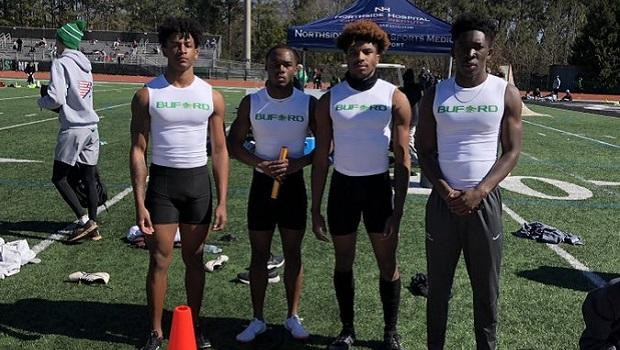# 2021 Outdoor Track: 6A HS Boys Team Power RankingsThe Buford boys 4x200 squad. (photo Marc Logan)

As per the end of Week 6 of the outdoor track season, there are five schools that are a cut above the others. Westlake remains on top by six points over Alexander, and within striking distance are Buford, Carrollton, and Shiloh.

### 2021 ga HS M Outdoor Track Team Scores

Calculated as of March 22, 2021

These rankings were calculated by scoring the rankings as a meet. We took the individual rankings and relay rankings and scored them as a normal meet: 10-8-6-5-4-3-2-1.

 1. Westlake 65 Bryce Southerland (110H 5 = 5) Daquan Tate (400m 6 = 6) Malik Mixon (110H 10 + 300H 10 + 400m 10 = 30) Relay Team (15) Terez Davis-Giles (400m 3 + 800m 6 = 9) 2. Alexander 59 Amir Dayal (800m 10 = 10) Brandon Benton (HJ 10 = 10) Cameron Yates (800m 4 = 4) Grant Crabtree (3200m 8 = 8) La'eeq Mahdi (TJ 2 = 2) Marcus Guidry (800m 3 = 3) Relay Team (20) Tavis Maloy (200m 2 = 2) 3. Buford High School 54.5 Isaiah Bond (100m 9 + 200m 8 = 17) Jaylon Taylor (HJ 3.5 = 3.5) Mack Green (D 4 = 4) Malik Williams (LJ 4.5 = 4.5) Relay Team (18) Tobi Olawole (200m 5.5 = 5.5) Victor Payne (110H 2 = 2) 4. Carrollton High School 49.43 Amare Hall (HJ 0.43 + TJ 1 = 1.43) Collin Jones (3200m 3 + 800m 8 = 11) David Johns (110H 1 = 1) Grant Briscoe (PV 10 = 10) Justin Parham (800m 5 = 5) Kareem Nazim (PV 6 = 6) Mike Higgins (HJ 5 = 5) Relay Team (4) Zaylen Cook (110H 4 + 300H 2 = 6) 5. Shiloh High School 46 Anthony Sheppard III (TJ 3 = 3) George Benjamin (110H 6 = 6) Joshua Scott (400m 1 = 1) Nathan Solomon (1600m 3 + 3200m 4 = 7) Patrez Thomas (300H 8 + 400m 2 = 10) Relay Team (19) 6. Heritage High School 32 Anthony Peters (S 1 = 1) Jalen Giles (TJ 10 = 10) Rashun Fountain (100m 1 + 200m 10 + 400m 4 + LJ 6 = 21) 7. Brunswick 31.43 Devin Smith (LJ 1 = 1) Devon Cummings (110H 3 = 3) Izaiah Butler (HJ 0.43 = 0.43) Nick Gray (110H 8 = 8) Relay Team (4) Riyon Rankin (HJ 7 = 7) Travis Massey (100m 5 = 5) Zebulun Jackson (100m 3 = 3) 8. Dacula 28 Adam Watkins (D 10 + S 10 = 20) Ben Butcher (1600m 4 + 3200m 2 = 6) Tyler Farris (PV 2 = 2) 9. Richmond Hill 25.5 Evan Clark (LJ 4.5 + TJ 4 = 8.5) Jasai Taylor (300H 6 = 6) Relay Team (11) 10. Rome High School 25.43 Connor Johnson (PV 5 = 5) Jay Wise (TJ 8 = 8) Martel Hight (HJ 0.43 = 0.43) Patrick Motes (1600m 1 + 3200m 10 + 800m 1 = 12) 10. Pope High School 25.43 Piers Cameron (D 8 + S 2 = 10) Relay Team (3) Theron Nixon (HJ 0.43 = 0.43) Zach Marinko (HJ 7 + TJ 5 = 12) 12. Allatoona HS 24.5 Eric Young (200m 5.5 + 400m 8 = 13.5) Griffin Reeve (PV 1 = 1) Levi Brown (LJ 8 = 8) Relay Team (2) 13. East Paulding High School 21 Ashlund Dixon (100m 9 + 200m 4 = 13) Lennie Hall (LJ 3 = 3) Marcus Ford (300H 5 = 5) 14. Evans High School 17 Hayden Rourke (D 1 = 1) Jonathan Greene (1600m 10 + 3200m 6 = 16) 15. Langston Hughes HS 16 Relay Team (2) Robert CJ Lockhart (100m 6 = 6) Xavier Smith (200m 3 + 400m 5 = 8) 15. Tucker 16 Iriq Moore (D 6 + S 4 = 10) Relay Team (6) 17. North Atlanta 15 Ethan Curnow (1600m 6 = 6) Relay Team (8) Syre Stewart (200m 1 = 1) 18. Glynn Academy 13.5 Terrance Lewis (LJ 10 = 10) Tyson Rooks (HJ 3.5 = 3.5) 19. South Effingham High School 13.43 Alexander Chambless (D 3 = 3) Cameron Edwards (HJ 0.43 + LJ 2 + TJ 6 = 8.43) Jake Kerns (D 2 = 2) 20. Habersham Central High School 10.86 Andrew Kivett (1600m 8 + 800m 2 = 10) Issac Simmons (HJ 0.43 = 0.43) Luke Cooper (HJ 0.43 = 0.43) 21. Winder-Barrow HS 8.5 Antonio Bishop (300H 3 = 3) Emorie Foskey (S 5.5 = 5.5) 22. Alcovy 8 Duvall Hill (S 8 = 8) 22. Riverwood International Charter School 8 Nick West (PV 8 = 8) 22. Osborne High School 8 Zyler Johnson (D 5 + S 3 = 8) 22. Cambridge HS 8 Caelan Jones (300H 4 = 4) Eric Garguilo (PV 4 = 4) 26. Chattahoochee High School 6 Diego Santiago (1600m 5 + 3200m 1 = 6) 27. Douglas County 5.5 Julian Dailey (S 5.5 = 5.5) 28. Creekview 5 Bryson Gates (3200m 5 = 5) 29. Bradwell Institute 4 Devin Nash (100m 4 = 4) 30. Sequoyah High School 3 Alex Kendall (PV 3 = 3) 30. Lakeside, Evans 3 Ethan Kurilko (1600m 2 = 2) Relay Team (1) 30. Valdosta High School 3 Art'xavian Williams (100m 2 = 2) Christopher Wolfe (300H 1 = 1) 30. Rockdale Co. 3 Relay Team (3) 34. River Ridge HS 1 Relay Team (1)